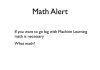Data Science

# The Mathematics of Machine LearningBy Wale Akinfaderin, PhD candidate in Physics at Florida State University

In the last few months, I have had several people contact me about their enthusiasm for venturing into the world of data science and using Machine Learning (ML) techniques to probe statistical regularities and build impeccable data-driven products. However, I’ve observed that some actually lack the necessary mathematical intuition and framework to get useful results. This is the main reason I decided to write this blog post.

Recently, there has been an upsurge in the availability of many easy-to-use machine and deep learning packages such as scikit-learn, Weka, Tensorflow etc. Machine Learning theory is a field that intersects statistical, probabilistic, computer science and algorithmic aspects arising from learning iteratively from data and finding hidden insights which can be used to build intelligent applications. Despite the immense possibilities of Machine and Deep Learning, a thorough mathematical understanding of many of these techniques is necessary for a good grasp of the inner workings of the algorithms and getting good results.

##### WHY WORRY ABOUT THE MATHS?

There are many reasons why the mathematics of Machine Learning is important and I’ll highlight some of them below:

1. Selecting the right algorithm which includes giving considerations to accuracy, training time, model complexity, number of parameters and number of features.

2. Choosing parameter settings and validation strategies.

3. Identifying underfitting and overfitting by understanding the Bias-Variance tradeoff.

4. Estimating the right confidence interval and uncertainty.

##### WHAT LEVEL OF MATHS DO YOU NEED?

The main question when trying to understand an interdisciplinary field such as Machine Learning is the amount of maths necessary and the level of maths needed to understand these techniques. The answer to this question is multidimensional and depends on the level and interest of the individual. Research in mathematical formulations and theoretical advancement of Machine Learning is ongoing and some researchers are working on more advance techniques. I’ll state what I believe to be the minimum level of mathematics needed to be a Machine Learning Scientist/Engineer and the importance of each mathematical concept.

1. Linear Algebra: A colleague, Skyler Speakman, recently said that “Linear Algebra is the mathematics of the 21st century” and I totally agree with the statement. In ML, Linear Algebra comes up everywhere. Topics such as Principal Component Analysis (PCA), Singular Value Decomposition (SVD), Eigendecomposition of a matrix, LU Decomposition, QR Decomposition/Factorization, Symmetric Matrices, Orthogonalization & Orthonormalization, Matrix Operations, Projections, Eigenvalues & Eigenvectors, Vector Spaces and Norms are needed for understanding the optimization methods used for machine learning. The amazing thing about Linear Algebra is that there are so many online resources. I have always said that the traditional classroom is dying because of the vast amount of resources available on the internet. My favorite Linear Algebra course is the one offered by MIT Courseware (Prof. Gilbert Strang).

2. Probability Theory and Statistics: Machine Learning and Statistics aren’t very different fields. Actually, someone recently defined Machine Learning as ‘doing statistics on a Mac’. Some of the fundamental Statistical and Probability Theory needed for ML are Combinatorics, Probability Rules & Axioms, Bayes’ Theorem, Random Variables, Variance and Expectation, Conditional and Joint Distributions, Standard Distributions (Bernoulli, Binomial, Multinomial, Uniform and Gaussian), Moment Generating Functions, Maximum Likelihood Estimation (MLE), Prior and Posterior, Maximum a Posteriori Estimation (MAP) and Sampling Methods.

3. Multivariate Calculus: Some of the necessary topics include Differential and Integral Calculus, Partial Derivatives, Vector-Values Functions, Directional Gradient, Hessian, Jacobian, Laplacian and Lagragian Distribution.

4. Algorithms and Complex Optimizations: This is important for understanding the computational efficiency and scalability of our Machine Learning Algorithm and for exploiting sparsity in our datasets. Knowledge of data structures (Binary Trees, Hashing, Heap, Stack etc), Dynamic Programming, Randomized & Sublinear Algorithm, Graphs, Gradient/Stochastic Descents and Primal-Dual methods are needed.

5. Others: This comprises of other Math topics not covered in the four major areas described above. They include Real and Complex Analysis (Sets and Sequences, Topology, Metric Spaces, Single-Valued and Continuous Functions, Limits), Information Theory (Entropy, Information Gain), Function Spaces and Manifolds.

Read the source post at Dataconomy.